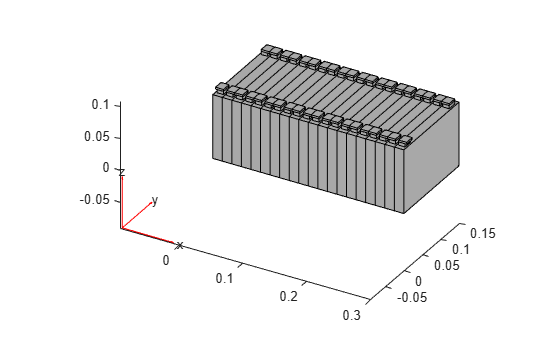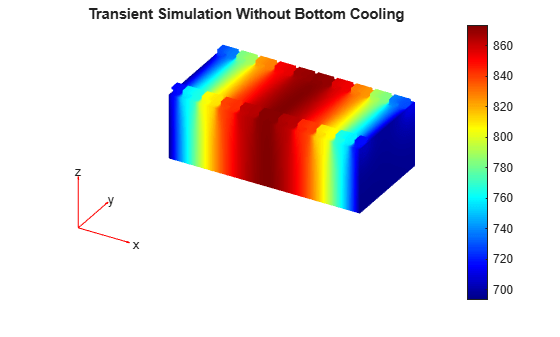# Battery Module Cooling Analysis and Reduced-Order Thermal Model

This example shows how to perform a battery cooling analysis determine the effect of a cooling system. In addition, this example generates a reduced-order model (ROM) for the battery module. You can use the resulting ROM in a system-level model in Simscape™.

### Battery Module Geometry

Define the key geometric parameters of a prismatic battery cell.

```cellWidth = 150/1000; cellThickness = 15/1000; tabThickness = 10/1000; tabWidth = 15/1000; cellHeight = 100/1000; tabHeight = 5/1000; connectorHeight = 3/1000;```

Define the number of cells in the battery module.

`numCellsInModule = 20;`

Create the geometry of the battery module by using the supporting function `createBatteryModuleGeometry`, which is provided in a supporting file.

```[geomModule,volumeIDs,boundaryIDs,volume,area,ReferencePoint] = ... createBatteryModuleGeometry(numCellsInModule, ... cellWidth, ... cellThickness, ... tabThickness, ... tabWidth, ... cellHeight, ... tabHeight, ... connectorHeight);```

Plot the geometry.

`pdegplot(geomModule)`The `createBatteryModuleGeometry` function returns geometric IDs required for assigning material properties and boundary conditions as two structure arrays, `volumeIDs` and `boundaryIDs`. Each structure array is organized by cell. The `volumeIDs` structure array contains five volume regions for each cell: the cell body, two tabs, and two connectors. The `boundaryIDs` structure array contains face IDs for the six faces of the cell body.### Transient Thermal Analysis

Create a thermal model for transient analysis, and assign the battery module geometry to the model.

```model = createpde("thermal","transient"); model.Geometry = geomModule;```

Generate and plot the mesh.

```generateMesh(model); pdemesh(model)```Collect IDs for assigning material properties and boundary conditions.

```cellIDs = [volumeIDs.Cell]; tabIDs = [volumeIDs.TabLeft,volumeIDs.TabRight]; connectorIDs = [volumeIDs.ConnectorLeft,volumeIDs.ConnectorRight]; bottomPlateFaces = [boundaryIDs.BottomFace];```

Specify the thermal conductivity of the battery, in W/(K*m).

```cellThermalCond.inPlane = 80; cellThermalCond.throughPlane = 2; tabThermalCond = 386; connectorThermalCond = 400;```

Specify the mass densities of the battery components, in kg/m³.

```density.Cell = 780; density.TabLeft = 2700; density.TabRight = 2700; density.ConnectorLeft = 540; density.ConnectorRight = 540;```

Specify the specific heat values of the battery components, in J/(kg*K).

```spHeat.Cell = 785; spHeat.TabLeft = 890; spHeat.TabRight = 890; spHeat.ConnectorLeft = 840; spHeat.ConnectorRight = 840;```

Assign the thermal material properties of the cell body, tabs, and connectors.

```thermalProperties(model,Cell=cellIDs, ... ThermalConductivity=[cellThermalCond.throughPlane cellThermalCond.inPlane cellThermalCond.inPlane], ... MassDensity=density.Cell, ... SpecificHeat=spHeat.Cell); thermalProperties(model,Cell=tabIDs, ... ThermalConductivity=tabThermalCond, ... MassDensity=density.TabLeft, ... SpecificHeat=spHeat.TabLeft); thermalProperties(model,Cell=connectorIDs, ... ThermalConductivity=connectorThermalCond, ... MassDensity=density.ConnectorLeft, ... SpecificHeat=spHeat.ConnectorLeft);```

Define the ambient temperature, in K.

`Tambient = 293;`

First, simulate the module with only natural convection cooling applied at the front and back faces of the module.

```thermalBC(model, ... Face=[boundaryIDs(1).FrontFace,boundaryIDs(end).BackFace], ... ConvectionCoefficient=15, ... AmbientTemperature=Tambient);```

Apply nominal heat generation. Assume that the heat generation during normal operation is 15 W.

```nominalHeatGen = 15/volume(1).Cell; internalHeatSource(model,nominalHeatGen,Cell=cellIDs);```

For a faulty cell, specify a higher heat generation value, for example, 25 W.

```faultCellHeatGen = 25/volume(10).Cell; internalHeatSource(model,faultCellHeatGen,Cell=volumeIDs(10).Cell);```

Specify the initial conditions, and solve the problem for 2 hours of operation.

```thermalIC(model,Tambient); R = solve(model,0:60:7200);```

Plot the temperature at the end of the simulation.

```pdeplot3D(model.Mesh,ColorMapData=R.Temperature(:,end)) title("Transient Simulation Without Bottom Cooling")```Include the cooling effect on the bottom faces of the module, and solve the problem again.

```thermalBC(model,Face=bottomPlateFaces, ... ConvectionCoefficient=100, ... AmbientTemperature=Tambient); R = solve(model,0:60:7200);```

Plot the temperature at the end of the simulation with bottom cooling.

```figure pdeplot3D(model.Mesh,ColorMapData=R.Temperature(:,end)) title("Transient Simulation with Bottom Cooling")```### Modal Analysis and Reduced-Order Model

Switch the analysis type of the thermal model to `"modal"`.

`model.AnalysisType = "modal";`

Solve for modes of the thermal model in the specified decay range.

`Rm = solve(model,DecayRange=[-Inf,5e-2]);`

Plot the mode shape for the first mode.

```figure pdeplot3D(model.Mesh,ColorMapData=Rm.ModeShapes(:,1)) title("Mode 1")```Use the modal results to reduce the thermal model.

`rom = reduce(model,ModalResults=Rm);`

The output `rom` contains a smaller-sized system to use in Simscape system-level modeling. In addition to the ROM, defining a control loop requires other data to couple the ROM with Simscape elements. The following sections create all the relevant data and populate a `pde_rom` structure array with it.

The Simscape Battery™ loop computes the heat generation loads and boundary loss. To apply these loads and losses on the ROM, use unit load vectors and scale them by using scaling factors calculated from the Simscape Battery libraries. These full-length scaled load vectors with a size equal to the finite-element model degrees of freedom (DoFs) are projected to reduce load to the ROM space by using the transformation matrix available in the ROM. The reduced load vector drives the ROM dynamics.

Generate load matrices corresponding to heat generation by using the `sscv_unitHeatLoadBatteryROM` and `sscv_unitBCBatteryROM` helper functions. The helper functions use `assembleFEMatrices` to get each source load matrix.

```heatGenUnit_cells = ... sscv_unitHeatLoadBatteryROM(model,cellIDs); heatGenUnit_tabs = ... sscv_unitHeatLoadBatteryROM(model,tabIDs'); heatGenUnit_connector = ... sscv_unitHeatLoadBatteryROM(model,connectorIDs'); heatLoadUnit_bottomFaces = ... sscv_unitBCBatteryROM(model,bottomPlateFaces);```

### Compute Lumped Thermal Mass of the Cell

Define the cell thermal mass required in the Battery (Table-Based) library component.

```cellThermalMass = zeros(1,numCellsInModule); for domain = ["Cell","TabLeft","TabRight", ... "ConnectorLeft","ConnectorRight"] cellThermalMass = cellThermalMass + ... [volume.(domain)].*[density.(domain)].*[spHeat.(domain)]; end```

### Thermocouple Locations to Probe Control Temperature

The thermocouples are attached to the center of the top surface of the cells. Define spatial coordinates of thermocouples by using reference point coordinates, for the center of the cells.

```refPointTopFaceCell1 = ... ReferencePoint.Cell + [0,0,cellHeight/2]; thermocouples.probe_locations = ... repmat(refPointTopFaceCell1,numCellsInModule,1); thermocouples.probe_locations(:,1) = ... ReferencePoint.Cell(1):-cellThickness:0;```

Use these coordinates to find the associated nearest node IDs of the finite-element mesh.

```thermocouples.probeNodeIDs = ... model.Mesh.findNodes("nearest",thermocouples.probe_locations'); thermocouples.numOfTempProbes = ... numel(thermocouples.probeNodeIDs);```

The ROM solution is defined using the reduced DoFs. To recover the solution in terms of physical temperatures DoFs, use the function `reconstructSolution`. For plotting purposes, create a submatrix `W` of `rom.TransformationMatrix` that can be used to generate the temperature-time history for the nodes corresponding to thermocouple locations.

```thermocouples.W = ... rom.TransformationMatrix(thermocouples.probeNodeIDs,:);```

### Array with Data Required for Simscape Model

Create the `pde_rom` structure array with all the data needed for a Simscape model. First, add the initial temperature of the battery, in K.

`pde_rom.prop.initialTemperature = Tambient;`

```pde_rom.numCellsInModule = numCellsInModule; pde_rom.prop.cell_width_mm = cellWidth; pde_rom.prop.cell_thickness_mm = cellThickness; pde_rom.prop.cell_height_mm = cellHeight; pde_rom.prop.tabWidth_mm = tabWidth; pde_rom.prop.tabThickness_mm = tabThickness; pde_rom.prop.connectorHeight_mm = connectorHeight; pde_rom.Geometry = model.Geometry; pde_rom.volume = volume; pde_rom.area = area;```

```pde_rom.prop.cellThermalCond.inPlane = ... cellThermalCond.inPlane; pde_rom.prop.cellThermalCond.throughPlane = ... cellThermalCond.throughPlane; pde_rom.prop.tabThermalCond = ... tabThermalCond; pde_rom.prop.connectorThermalCond = ... connectorThermalCond; pde_rom.prop.density = ... density; pde_rom.prop.spHeat = ... spHeat; pde_rom.prop.cellThermalMass = ... cellThermalMass; ```

Add the thermal load matrices. The `heatGenUnit_cells` and `heatLoadUnit_bottomFaces` fields contain load matrices, with each column of a matrix corresponding to a cell in the module. The `heatGenUnit_tabs` and `heatGenUnit_connector` fields contain load vectors for both the positive and negative ends for each cell.

```pde_rom.Q.heatGenUnit_cells = ... sscv_unitHeatLoadBatteryROM(model,cellIDs); pde_rom.Q.heatGenUnit_tabs = ... sscv_unitHeatLoadBatteryROM(model,tabIDs'); pde_rom.Q.heatGenUnit_connector = ... sscv_unitHeatLoadBatteryROM(model,connectorIDs'); pde_rom.Q.heatLoadUnit_bottomFaces = ... sscv_unitBCBatteryROM(model,bottomPlateFaces);```

`pde_rom.thermocouples = thermocouples;`

`pde_rom.rom = rom;`

Save the structure array to a MAT-file.

`save batteryModuleThermalROM pde_rom`

### Helper Functions

Assemble the load vector due to the volumetric heat generation.

```function F = sscv_unitHeatLoadBatteryROM(model,domainIDs) % Assign zero globally F = zeros(size(model.Mesh.Nodes,2),numel(domainIDs)); heatsourceHandle = internalHeatSource(model,1,Cell=1); for i = 1:numel(domainIDs) % Assign unit heat generation on specified domain heatsourceHandle.RegionID = domainIDs(i); % Assemble the load vector mats = assembleFEMatrices(model,"F"); F(:,i) = mats.F; end end ```

Assemble the load vector due to the boundary heat flux.

```function G = sscv_unitBCBatteryROM(model,boundaryIDs) % Assign zero globally. G = zeros(size(model.Mesh.Nodes,2),numel(boundaryIDs)); bchandle = thermalBC(model,HeatFlux=-1,Face=1); for i = 1:numel(boundaryIDs) % Assign negative heat flux specified boundary bchandle.RegionID = boundaryIDs(i); % Assemble the load vector mats = assembleFEMatrices(model,"G"); G(:,i) = mats.G; end end ```